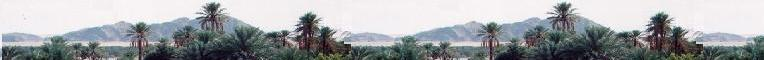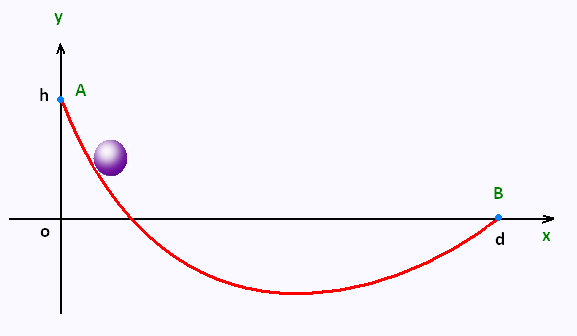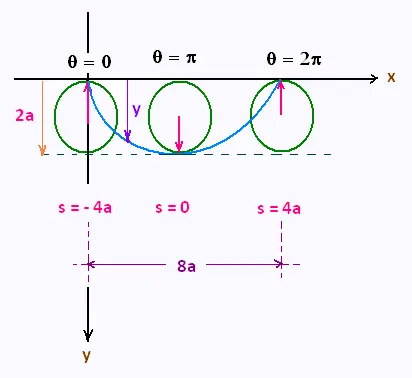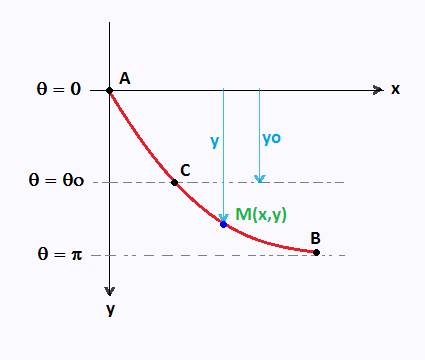###### Variational Methods

Contents

Euler Lagrange Equation

Lagrangian Mechanics

Application

# Calculus of Variations: Functionals Principle of Least Action The shortest path The brachistochrone curve

### 1. The brachistochrone curve

The brachistochrone curve or , im Mathematics, the cycloid is the curve of the «quickest descent» that undergoes a point-like body, starting at rest and moving along this curve, without friction, under constant gravity, to a given end point in the shortest time.Let's choose two points A(xA = 0, yA = h) and B(xB = d, yB = 0) that are not directly above each other, and join them with a wire.

Suppose we allow a bead to slide down the wire, witout initial velocity, under the influence of gravity (ignoring friction).

Of all possible shapes that the wire can be bent into, the bead will slide from A to B, the fastest, when the shape is half of an arch of a cycloid , or an inverted cycloid.

The brachistochrone curve is also the curve of «equal descent» in the sense that no matter where we place a bead on a cycloid-shaped wire, it takes the same time to slide to the bottom.

Conclusion:

The shortest path, between two points , under the effect of gravity is a cycloid.

### 2. Equations of the brachistochrone curve

We will first express the travel time between A and B, then look for the curve, that is the shape of the slide, that minimizes this time.

Let s the curvilinear abscissa. An element ds of the curve is expressed in cartesian coordinates as

ds = [1 + (dy/dx)2]1/2 dx = [1 + y'2]1/2 dx

ds/dx = [1 + (dy/dx)2]1/2 dx = [1 + y'2]1/2

The speed of the object is given by v = ds/dt.

The conservation of the mechanical energy at a point (x,y) is written as:

(1/2)mv2 + mgy = mgh

That gives:

v = [2g(h - y)]1/2

v = ds/dt = [2g(h - y)]1/2

Let express the travel time T[y]. It is a functional that depends on the function y(x) of the bead. We have

dx/ds = (dx/dt) (dt/ds).

Therefore

dt/dx = (dt/ds)/(dx/ds) = (1/v)/(dx/ds).

Then

dt = (1/v) (ds/dx) dx = [1 + y'2]1/2/[2g(h - y)]1/2 dx
= [(1 + y'2)/2g(h - y)]1/2

dt = [(1 + y'2)/2g(h - y)]1/2 dx

The travel time T[y] is then written :

T[y] = 0d f(y, y')dx

Where f(y,y') = [(1 + y'2)/2g(h - y)]1/2.
f(y,y') does not depend on x.

We search them te function y(x) that minimizes the fonctional T[y].

Since f does not depend on x, we can then use the Euler-Lagrange simplified (Beltrami formula):

f - y'(∂f/∂y') = constant.

Let constant = C1. First, let's calculate the partial derivative ∂f/∂y':

∂f/∂y' = (1/2) (1 + y'2)-1/2 2y'/[2g(h - y)]1/2 =
y'/[2g(h - y) (1 + y'2)]1/2

∂f/∂y' = y'/[2g(h - y) (1 + y'2)]1/2

Then:

f - y'(∂f/∂y') = (1 + y'2)/2g(h - y)1/2 - y'2/[2g(h - y) (1 + y'2)]1/2 =
(1 + y'2)1/2 - y'2/(1 + y'2)1/2 = C1 . [2g(h - y)]1/2

Hence:

1/(1 + y'2)1/2 = C1. [2g(h - y)]1/2

Squaring, the two members of the equality, we obtain:

1 + y'2 = C2 /2g(h - y) = C2/(h - y) , with C2 = C12

Let the constant R = C2/2g, then

1 + y'2 = R/(h - y)

Let y' = tan(θ/2). So 1 + y'2 = 1 + tan2 (θ/2) = 1/cos2(θ/2) = R /(h - y)

Therefore

y = h - R cos2 (θ/2) = h - (R/2)(1 + cos θ)

with dy/dθ = (R/2) sin θ

y' = tan (θ/2) = dy/dx = (dy/dθ)(dθ/dx) = (R/2) sin θ . (dθ/dx)

Then :

tan (θ/2) = (R/2) sin θ . (dθ/dx)

sin (θ/2) /cos (θ/2) = 2 (R/2) sin(θ/2) . cos(θ/2) . (dθ/dx)

1 /cos (θ/2) = R cos(θ/2) . (dθ/dx)

Therefore:

dx = 1 /cos (θ/2) = R cos2(θ/2) . (dθ) = (R/2) (1 + cos θ) dθ

dx = (R/2) (1 + cos θ) dθ .

Its integral is x = (R/2) (θ + sin θ) + sct

We have then:

x = (R/2) (θ + sin θ) + cst
y = h - (R/2)(1 + cos θ)

These are the equations of a cycloid.

With the change of the variable θ as θ --> θ - π
and the condition x(0) = 0 , these equations become:

x = (R/2) (θ - sin θ)
y = h - (R/2)(1 - cos θ)

We can make other conditions, by requiring that the curve pass through the point B(XB, yB), to fix the constant R.

We can also take h = 0 and direct the y axis to - y axis, and end with the two following simple equations:

x = a (θ - sin θ)
y = a (1 - cos θ)

### 2. Equations of the motion of the beard on tyhe cycloid using the LagrangianThe coordinates (x,y) of any point on the cycloid are:

x = a (θ - sin θ)
y = a (1 - cos θ)

The correspondant derivative are:

dx = a(1 - cos θ)dθ
dy = a sin θ dθ

The squared length of a curvilinear element ds is:

ds2 = dx2 + dy2 = a2 [(1 - cos θ)2 + sin2θ] (dθ)2 =

2 a2 (1 - cos θ) (dθ)2 = 4 a2 sin2(θ/2) (dθ)2

So ds = 2a sin(θ/2) (dθ)

ds = 2a sin(θ/2) (dθ)

Let the displacement s mesured, along the cycloid, from the bottom at &theta = π. The integration gives:

s = ∫πθ2a sin(θ/2) (dθ) = - 4 a cos(θ/2)

s = - 4 a cos(θ/2)

The displacement s ranges from s(0) = - 4a to s(2π) = 4 a.

The kinetic energy of the bead of mass m which slides along the cycloid is :

T = (1/2) m (ds/dt)2

T = (m/2) (ds/dt)2

The gravitational potential energy of the bead , mesured from the bottom of the cycloid is:

V = mg(2a - y) = mg( 2a - a (1 - cos θ)) = mga (1 + cos θ) =
2 m g a cos2 (θ/2) = (1/2) m (g/4a) s2

V = (m/2) (g/4a) s2

The lagrangian is :

L = T - V = (m/2) (ds/dt)2 - (m/2)(g/4a) s2

L = (m/2) [(ds/dt)2 - (g/4a) s2]

The lagrage's equation, with v = ds/dt, is written as:

d[∂L/∂v]/dt = ∂L/∂s .

We have: :

∂L/∂v = (m/2) 2 (ds/dt). So
d[∂L/∂v] = m d2s/dt2, and
∂L/∂s = (m/2)2 (- (g/4a) s) = - m (g/4a) s

Thus

d2s/dt2 + (g/4a) s = 0

d2s/dt2 + (g/4a) s = 0

This is the equation of motion of a single harmonic oscillator with angular frequency ω = √(g/4a) and period T = 2π/ω = 4π √(a/g).

### 3. Expression of the unique time independent of the starting pointThe time to fall from the point A to the point B on the cycloid is independent of the starting point A.

We the following two parametric equations of the cycloid:

x = a(θ - sin θ)
y = a(1 - cos θ)

The correspondant derivative are:

dx = a(1 - cosθ) dθ
dy = a sin θ dθ

so

ds2 = dx2 + dy2 = 2a2 (1 - cos θ) (dθ)2

The origine of potential energy at y = 0:

At any point M(x, y) on the curve, using the concervation of energy law , we obtain

(1/2) m v2 = m g y

Since v = ds/dt,
dt = ds/v = a (√(2(1 - cos θ)) dθ)/(√(2gy)) =
a (√(2(1 - cos θ)) dθ)/(√(2ga(1 - cosθ))) = √(a/g) dθ

dt = √(a/g) dθ

Therefore, the time to travel to the point A(θ = 0) to the point B(θ = π) is

T = ∫0π dt = π √(a/g)

T = π √(a/g)

The origine of potential energy at y = yo:

At any other point, say M(x, Y = y - yo), we will have :

y = a(1 - cos θ)
yo = a(1 - cos θo)

y - yo = cos θo - cos θ

(1/2) m v2 = m g Y = m g (y - yo) = mga (cos θo - cos θ)

dt = ds/v = a (√(2(1 - cos θ)) dθ)/(√(2gY)) =
a (√(2(1 - cos θ)) dθ)/(√(2ga(cos θo - cos θ))) =
√(a/g) √ [(1 - cos θ)/(cos θo - cos θ)] dθ

dt = √(a/g) √ [(1 - cos θ)/(cos θo - cos θ)] dθ

Therefore, the time to travel to the point C(θ = θo)
to the point B(θ = π) is

T = ∫0π dt = √(a/g) ∫θoπ √ [(1 - cos θ)/(cos θo - cos θ)] dθ

Substituting the following trigonometric formulas:

cos (θ/2) = √[(1 + cos θ)/2],
sin (θ/2) = √[(1 - cos θ)/2],

The integral becomes:

T = ∫0π dt = √(a/g) ∫θoπ √ [(sin (θ/2))/(cos (θo/2) - cos (θ/2)] dθ

Using the following change of variables :

u = cos(θ/2)/cos(θo/2) ,so
du = - (1/2) dθ sin(θ/2)/cos(θo/2)

Therefore:

T = 2 √(a/g) ∫01 du/√(1 - u)2 = 2 √(a/g) [Arcsin (u)]01 = π √(a/g).

T = π √(a/g)

Conclusion:

The time is the same from any starting point in the cycloid.

 chimie labs | Physics and Measurements | Probability & Statistics | Combinatorics - Probability | Chimie | Optics | contact |